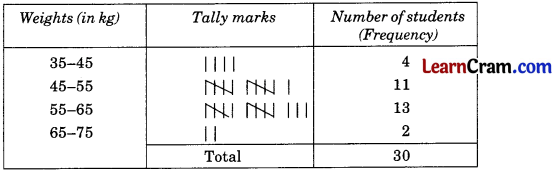# DAV Class 8 Maths Chapter 15 Worksheet 4 Solutions

The DAV Class 8 Maths Solution and DAV Class 8 Maths Chapter 15 Worksheet 4 Solutions of Statistics and Probability offer comprehensive answers to textbook questions.

## DAV Class 8 Maths Ch 15 WS 4 Solutions

Question 1.
You are given a spinning wheel. How many outcomes can you see?Solution:
Number of outcomes are A, B, C, D i.e. 4.

Question 2.
I have a well-shuffled deck of 52 playing cards. Write down the
probability of getting the following out of the deck of cards when a card drawn:
(i) an ace
Solution:
Here n(S) = 52
and n(E) = 4
Probability of getting an ace = $$\frac{4}{52}=\frac{1}{13}$$

(ii) a red 10
Solution:
Here n(S) = 52
and n(E) = 4
Probability of getting a red 10 = $$\frac{2}{52}=\frac{1}{26}$$

(iii) a diamond queen.
Solution:
Here n(S) = 52
and n(E) = 4
Probability of getting a diamond queen = $$\frac{1}{52}$$Question 3.
There are 25 chalks in a box out of which 8 are yellow chalks, 11 are blue chalks, and the rest are white chalks. You are asked to pick a chalk from the box without looking into the box. What is the probability of:
(i) getting a yellow chalk?
Solution:
Total number of chalks = 25
Number of yellow chalks = 8
∴ Probability of getting yellow chalk = $$\frac{8}{25}$$

(ii) getting a blue chalk?
Solution:
Total number of chalks = 25
Number of blue chalk = 11
∴ Probability of getting blue chalk = $$\frac{11}{25}$$

(iii) getting a white chalk?
Solution:
Total number of chalks = 25
Remaining chalks are 25 – (8 + 11) = 6
∴ Probability of getting white = $$\frac{6}{25}$$Question 4.
Letters of alphabet are written on 26 separate slips and put in a bag. One slip is chosen from the bag. What is the probability of:

(i) getting the letter Q?
Solution:
Here n(S) = 26
and n(E) = 1
∴ Probability of getting Q = $$\frac{n(\mathrm{E})}{n(\mathrm{~S})}=\frac{1}{26}$$

(ii) getting a vowel?
Solution:
Here n(S) = 26 and n(E) = 1
Vowels are a, e, i, o, u i.e. 5
∴ Probability of getting a vowel = $$\frac{5}{26}$$### DAV Class 8 Maths Chapter 15 Value Based Questions

Question 1.
A school organised a camp for training students for athletic meet. The condition for selection was that the weight of a student should be 45 – 55 kg. For this purpose, the weights of 30 students of Class-VIII were taken and recorded as follows (in kg:)

42, 56, 64, 47, 54, 66, 57, 58, 44, 50, 49, 59, 62, 70, 40, 56, 56, 56, 43, 47, 60, 63, 49, 54, 58, 45, 47, 49, 64, 54.

(i) Prepare a frequency distribution and draw a histogram for the above data.
(ii) In what weight range do most students lie?
(iii) How many students got selected for the training?
(iv) What percentage of students weigh more than 55 kg?
(v) What factors are responsible for so many children being overweight and obese these days?
(vi) How can healthy eating habits be developed amongst the students of this age group?
Solution:
(i) Frequency distribution table:Histogram:
Scale on x-axis: 1 unit = 10 kg
Scale on y-axis: 1 unit = 2 students(ii) In weight range 55 – 85, most students lie.
(iii) 11 students got selected for the training.
(iv) Number of students having weight more than 55 kg = 13 + 2 = 15
Total number of students = 30
Percentage of students having weight more than 55 kg = $$\frac{15}{30}$$ × 100 = 50%
(v) Many factors like eating habits, lack of physical fitness, etc. are responsible for so many children being overweight and obese these days.
(vi) By sensitising towards eating habits arid their effects, healthy eating habits can be developed amongst the students of their age groups.